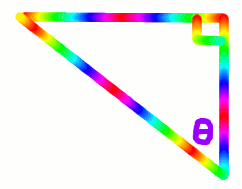# Introductory Trigonometry

How are heights of mountains measured? Mountain climbers do not carry tape measures. Mathematics called trigonometry is used. The word "trigonometry" has three parts - "tri" means "three", "gon" means "shape", and "metry" means "measure". We will use rules to calculate the side lengths and the angles of right-angled triangles.

## Parts of a Right-Angled TriangleRight angle - In a right-angled triangle, there is always a right angle or 90°.

Angle θ - the angle in which we are interested; uses the Greek letter "theta" which is the equivalent of the English letter "T"

Hypotenuse - the side opposite the right angle

Opposite side - the side opposite angle; the side all the way across the triangle from angle θ

Adjacent side - the side adjacent angle θ; the side next door to angle θ

## Did You Know That...?What is the world's highest mountain?

If you said Mount Everest in Nepal, you would be correct for the highest mountain above sea level.

If you said Mount Chimborazo in Ecuador (see picture above), you would be correct for the mountain furthest from the centre of the Earth.

## Example - Parts of a Right-Angled Triangle

In the following right triangle, which are the Hypotenuse, the Opposite side and the Adjacent side?

Mark these with H, O and A.## 3 Trigonometry Ratios or Rules

 Sin θ = Opposite side Hypotenuse
 Cos θ = Adjacent side Hypotenuse
 Tan θ = Opposite side Adjacent side

Where sin, cos and tan are abbreviations for mathematical operations called sine, cosine and tangent.

## Did You Know That...?

For brevity, the three trigonometry rules are abbreviated even further to:

• Sin θ = O/H
• Cos θ = A/H
• Tan θ = O/A

A mnemonic to remember these rules is "Some Old Hags Can't Always Hide Their Old Age" or "SOH-CAH-TOA".

## Question

To learn the three trigonometry rules, make up a mnemonic of your own.